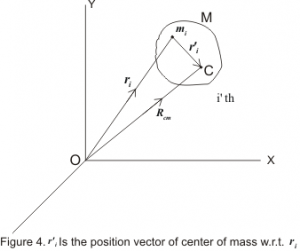# Kinetic energy of system of particles

This article is about Kinetic energy of system of particles. This topic comes under the chapter Dynamics of System of Particles. It is for B.Sc. students and comes under subject mechanics. For full chapter notes links
Dynamics of System of Particles

## Kinetic energy of the system of particles

• Let there are n number of particles in a n particle system and these particles possess some motion. The motion of the i’th particle of this system would depend on the external force Fi acting on it. Let at any time if the velocity of i’th particle be vi then its kinetic energy would be
\begin{equation*}
{E_{Ki}} = \frac{1}{2}mv_i^2
\end{equation*}
\begin{equation}
{E_{Ki}} = \frac{1}{2}m({{\rm{v}}_i}{\rm{.}}{{\rm{v}}_i}) \tag{1}
\end{equation}
• Let ri be the position vector of the i’th particle w.r.t. O and r’i be the position vector of the centre of mass w.r.t. ri ,as shown below in the figure , then
ri=r’i+Rcm                              (2)
where Rcm is the position vector of centre of mass of the system w.r.t. O.Differentiating equation 2 we get
\begin{equation*}
\frac{{d{{\rm{r}}_i}}}{{dt}} = \frac{{d{\rm{r}}{{\rm{‘}}_i}}}{{dt}} + \frac{{d{{\rm{R}}_{cm}}}}{{dt}}
\end{equation*}
or,
\begin{equation*}
{{\rm{v}}_i} = {{\rm{v}}_i}’ + {{\rm{V}}_{cm}} \tag{3}
\end{equation*}
where vi is the velocity of i’th particle w.r.t. centre of mass and Vcm is the velocity of centre of mass of system of particle. Putting equation 3 in 1 we get,
\begin{equation*}
{E_{Ki}} = \frac{1}{2}{m_i}\left[ {({{\text{v}}_i}^\prime + {{\text{V}}_{cm}}).({{\text{v}}_i}^\prime + {{\text{V}}_{cm}})} \right] = \frac{1}{2}{m_i}[({\text{v}}_{\text{i}}^{{{\text{2}}^\prime }} + 2{\text{v}}{{\text{‘}}_i}.{{\text{V}}_{{\text{cm}}}}{\text{ + V}}_{{\text{cm}}}^{\text{2}}){\text{]}}
\end{equation*}

\begin{equation*}
{E_{Ki}} = \frac{1}{2}{m_i}{\rm{v}}_{\rm{i}}^{{\rm{‘2}}} + {m_i}{\rm{v}}{{\rm{‘}}_i}{\rm{.}}{{\rm{V}}_{cm}} + \frac{1}{2}{m_i}{\rm{V}}_{{\rm{cm}}}^{\rm{2}} \tag{4}
\end{equation*}

• Sum of Kinetic energy of all the particles can be obtained from equation 4
$${E_K} = \sum\limits_{i = 1}^n {{E_{Ki}}} = \sum\limits_{i = 1}^n {\left[ {\frac{1}{2}{m_i}{\rm{v}}_{\rm{i}}^{{\rm{‘2}}} + {m_i}{\rm{v}}{{\rm{‘}}_i}{\rm{.}}{{\rm{V}}_{cm}} + \frac{1}{2}{m_i}{\rm{V}}_{{\rm{cm}}}^{\rm{2}}} \right]}$$
$${E_K} = \sum\limits_{i = 1}^n {\frac{1}{2}{m_i}{\rm{v}}_{\rm{i}}^{{\rm{‘2}}}} + \sum\limits_{i = 1}^n {{m_i}{\rm{v}}{{\rm{‘}}_i}{\rm{.}}{{\rm{V}}_{cm}}} + \sum\limits_{i = 1}^n {\frac{1}{2}{m_i}{\rm{V}}_{{\rm{cm}}}^{\rm{2}}}$$
$${E_K} = \frac{1}{2}{\rm{V}}_{{\rm{cm}}}^{\rm{2}}\sum\limits_{i = 1}^n {{m_i}} + \sum\limits_{i = 1}^n {\frac{1}{2}{m_i}{\rm{v}}_{\rm{i}}^{{\rm{‘2}}}} + {{\rm{V}}_{cm}}\sum\limits_{i = 1}^n {{m_i}{\rm{v}}{{\rm{‘}}_i}}$$
\begin{equation*}
{E_K} = \frac{1}{2}{\rm{V}}_{{\rm{cm}}}^{\rm{2}}M + \sum\limits_{i = 1}^n {\frac{1}{2}{m_i}{\rm{v}}_{\rm{i}}^{{\rm{‘2}}}} + {{\rm{V}}_{cm}}\frac{d}{{dt}}\sum\limits_{i = 1}^n {{m_i}{\rm{r}}{{\rm{‘}}_i}} \tag{5}
\end{equation*}
• Now last term in above equation which is
$\sum\limits_{i = 1}^n {{m_i}{{\rm{r’}}_{i}}} = 0$
would vanish as it defines the null vector because
$\sum\limits_{i = 1}^n {{m_i}{\rm{r}}{{\rm{‘}}_i}} = \sum\limits_{i = 1}^n {{m_i}({{\rm{r}}_i} – {{\rm{R}}_{cm}}) = M} {{\rm{R}}_{cm}} – M{{\rm{R}}_{cm}} = 0$
• Therefore kinetic energy of the system of particles is,
\begin{equation*}
E_K = \frac{1}{2}MV_{cm}^2 + \frac{1}{2}\sum\limits_{i = 1}^n {{m_i}{{v’_i}^2}} = E_{Kcm} + E_{K’} \tag{6}
\end{equation*}
where,
${E_{Kcm}} = \frac{1}{2}V_{{\rm{cm}}}^{\rm{2}}M$
is the kinetic energy obtained as if all the mass were concentrated at the center of mass and
$E{‘_K} = \sum\limits_{i = 1}^n {\frac{1}{2}{m_i}{\rm{v}}_{\rm{i}}^{{\rm{‘2}}}}$is the kinetic energy of the system of particle w.r.t. the centre of mass.
• Hence it is clear from equation 6 that kinetic energy of the system of particles consists of two parts: the kinetic energy obtained as if all the mass were concentrated at the centre of mass plus the kinetic energy of motion about the centre of mass.
• If there were no external force acting on the particle system then the velocity of the centre of mass of the system will remain constant and Kinetic Energy of the system would also remain constant.

#### Recommended texts on Dynamics of system of particles

http://www.astro.uwo.ca/~houde/courses/PDF%20files/physics350/Systems_Particles.pdf

http://teacher.pas.rochester.edu/phy121/lecturenotes/Chapter09/Chapter9.html

http://www.spumone.org/courses/dynamics-notes/sysparticles/

https://ocw.mit.edu/courses/mechanical-engineering/2-003j-dynamics-and-control-i-spring-2007/lecture-notes/lec05.pdf